# 【数学建模】数模day13-灰色系统理论I-灰色关联与GM(1,1)预测

0.

1.

1) 做关联分析首先讨论数据变换技术。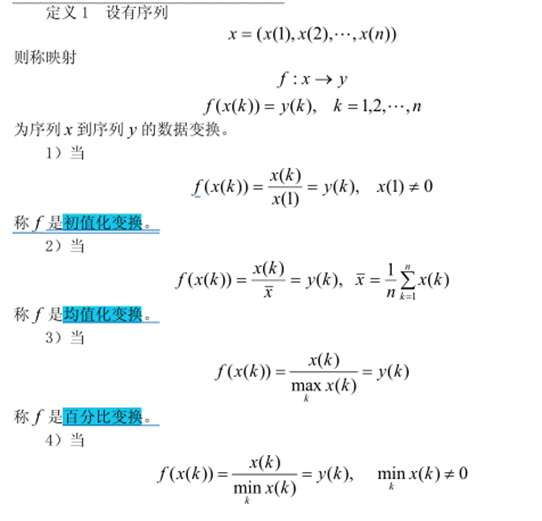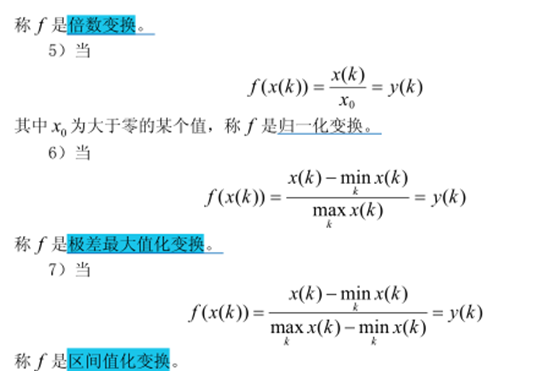2) 做关联分析：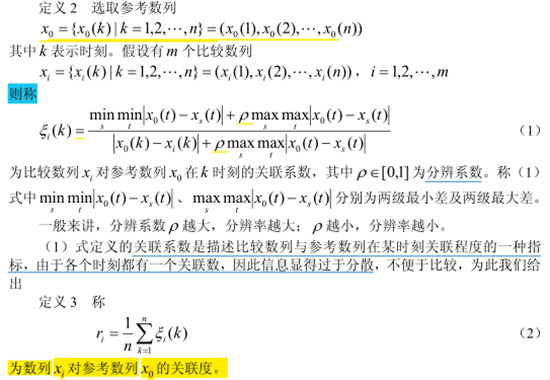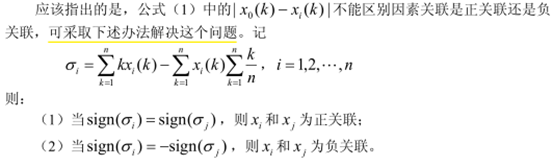3) 关联分析案例：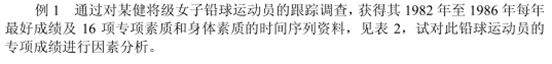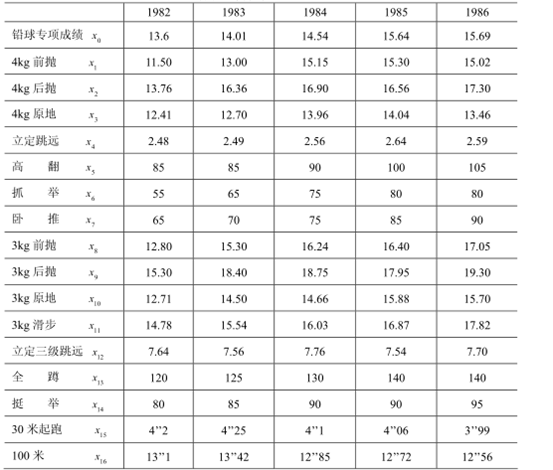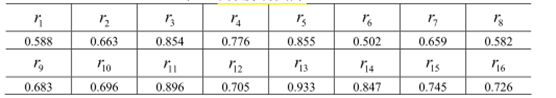matlab程序如下：

13.6  14.01  14.54  15.64  15.69
11.50  13.00  15.15  15.30  15.02
13.76  16.36  16.90  16.56  17.30
12.41  12.70  13.96  14.04  13.46
2.48  2.49  2.56  2.64  2.59
85  85  90  100  105
55  65  75  80  80
65  70  75  85  90
12.80  15.30  16.24  16.40  17.05
15.30  18.40  18.75  17.95  19.30
12.71  14.50  14.66  15.88  15.70
14.78  15.54  16.03  16.87  17.82
7.64  7.56  7.76  7.54  7.70
120  125  130  140  140
80  85  90  90  95
4.2  4.25  4.1  4.06  3.99
13.1  13.42  12.85  12.72  12.56

 1 % 关联分析
3  for i = 1:15
4      x(i,:) = x(i,:)/x(i,1); %前15数列做标准化
5 end
6  for i = 16:17
7      x(i,:) = x(i,1)./x(i,:); %后两个做标准化
8 end
9  data = x;
10  n = size(data,2); %矩阵列数，即观测时刻的个数
11 ck = data(1,:); %选第一列是参考数列
12 bj = data(2:end,:);%其余列是比较数列
13 m2 = size(bj,1);%比较数列个数
14 for j = 1:m2
15      t(j,:) = bj(j,:)-ck;
16  end
17  mn = min(min(abs(t'))); %最小差
18 mx = max(max(abs(t'))); %最大差
19 rho = 0.5; %分辨系数设置
20 ksi = (mn + rho*mx)./(abs(t)+rho*mx);%求关联系数
21 r = sum(ksi')/n %关联度
22 [rs,rind] = sort(r,'descend') %关联度排序‘

matlab结果：

r =

1 至 6 列

0.5881    0.6627    0.8536    0.7763    0.8549    0.5022

7 至 12 列

0.6592    0.5820    0.6831    0.6958    0.8955    0.7047

13 至 16 列

0.9334    0.8467    0.7454    0.7261

rs =

1 至 6 列

0.9334    0.8955    0.8549    0.8536    0.8467    0.7763

7 至 12 列

0.7454    0.7261    0.7047    0.6958    0.6831    0.6627

13 至 16 列

0.6592    0.5881    0.5820    0.5022

rind =

1 至 10 列

13    11     5     3    14     4    15    16    12    10

11 至 16 列

9     2     7     1     8     6

2.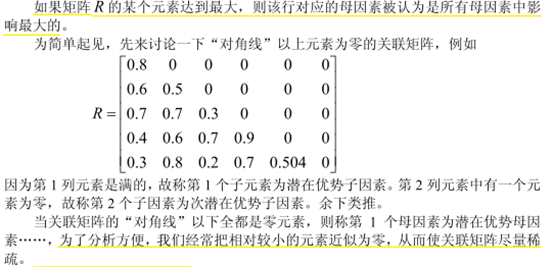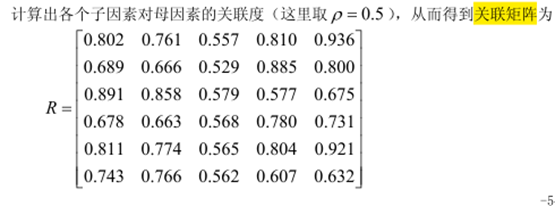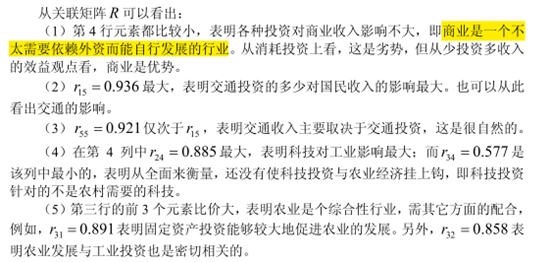3.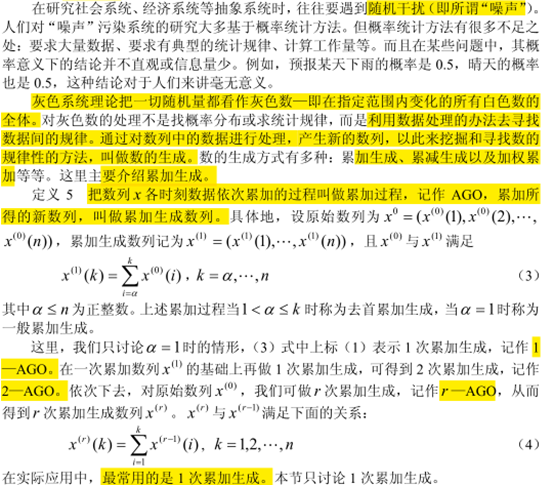4.

GM是Grey Model的简写。

1）GM(1,1)定义：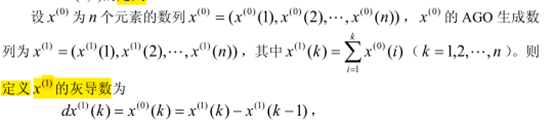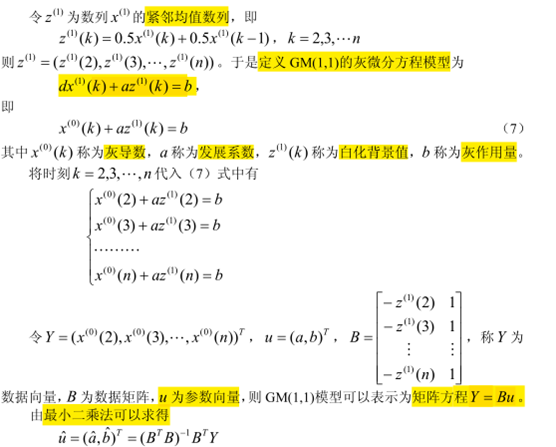2）GM(1,1)的白化型：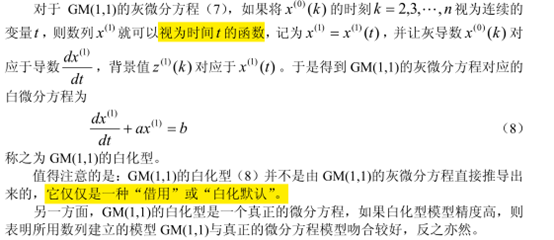5.

1) 灰色预测的方法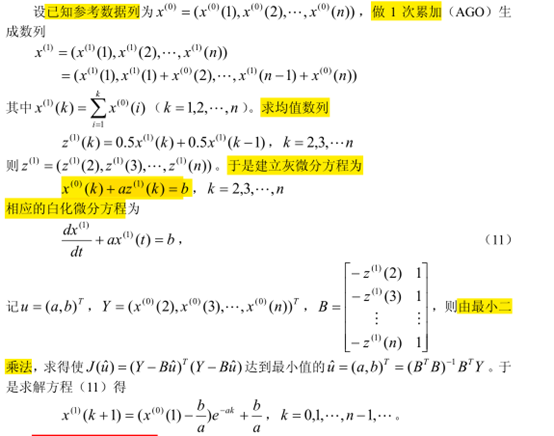2) 灰色预测处理的步骤(使用)

1. 数据的检验和处理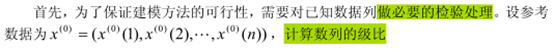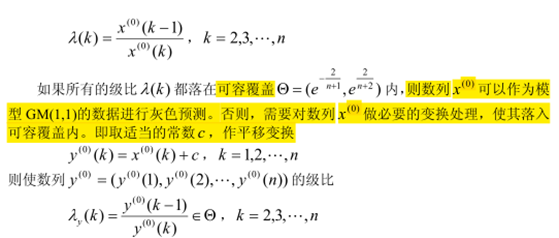2. 建立模型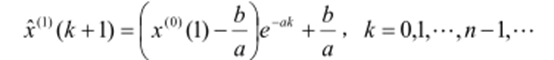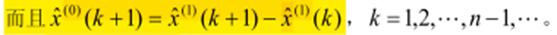3. 检验预测值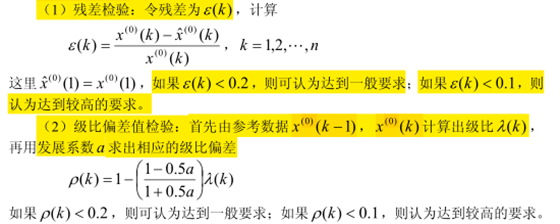4. 预测预报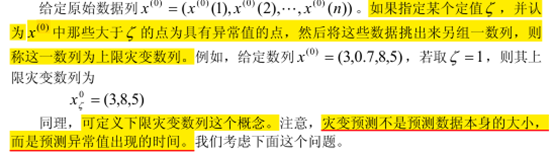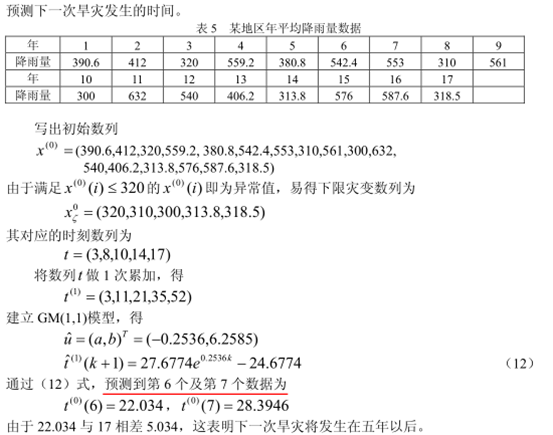6.

 步骤： 第一步：级比检验 (1)求级比，列出级比向量。 (2)级比判断：若所有级比都落在可容覆盖内，则通过，说明原始数据适合使用GM(1,1)   第二步：GM(1,1)建模 （1）原始数据做一次累加 （2）列出GM(1,1)模型 第三步：求解模型 （1）最小二乘法求解GM(1,1) （2）求生成数列预测值以及模型还原值 （3）相应可以得到预测值-真实值比较表格 第四步：模型检验 （1）原始值-模型值-残差-相对误差0级比偏差表格 （2）根据表格作出说明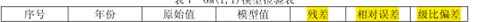序号 年份 Leq 1 1986 71.1 2 1987 72.4 3 1988 72.4 4 1989 72.1 5 1990 71.4 6 1991 72.0 7 1992 71.6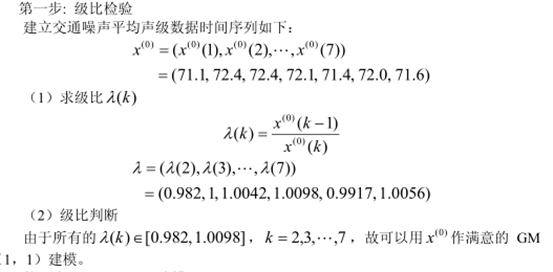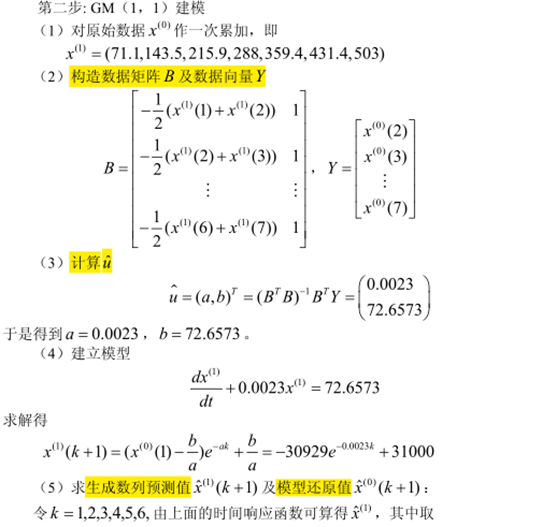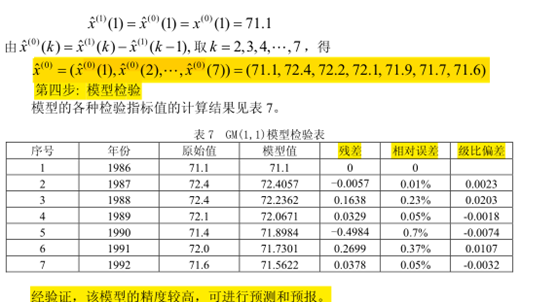%此程序原著pdf上程序是有bug的，以下已经调通

 1 clc,clear
2  x0=[71.1 72.4 72.4 72.1 71.4 72.0 71.6]';%注意这里为列向量
3 n=length(x0);
4  lamda=x0(1:n-1)./x0(2:n) %计算级比
5 range=minmax(lamda') %计算级比的范围
6 x1=cumsum(x0); %累加运算
7 B=[-0.5*(x1(1:n-1)+x1(2:n)),ones(n-1,1)];
8  Y=x0(2:n);
9  u=B\Y %最小二乘，拟合参数
10 syms x(t)
11  x=dsolve(diff(x)+u(1)*x==u(2),x(0)==x0(1));
12  x=subs(x,{'a','b','x0'},{u(1),u(2),x1(1)});
13  yuce1=subs(x,t,[0:n-1]);
14  yuce1 = double(yuce1);
15  %为提高预测精度，先计算预测值，再显示微分方程的解
16 y=vpa(x,6) %其中的 6 表示显示 6 位数字
17 yuce=[x0(1),diff(yuce1)] %差分运算，还原数据
18 epsilon=x0'-yuce %计算残差
19 delta=abs(epsilon./x0') %计算相对误差
20 rho=1-(1-0.5*u(1))/(1+0.5*u(1))*lamda' %计算级比偏差值

lamda =

0.9820
1.0000
1.0042
1.0098
0.9917
1.0056

range =

0.9820    1.0098

u =

0.0023
72.6573

y = 31000.0 - 30928.9*exp(-0.00234379*t)

yuce =

1 至 6 列

71.1000   72.4057   72.2362   72.0671   71.8984   71.7301

7 列

71.5622

epsilon =

1 至 6 列

0   -0.0057    0.1638    0.0329   -0.4984    0.2699

7 列

0.0378

delta =

1 至 6 列

0    0.0001    0.0023    0.0005    0.0070    0.0037

7 列

0.0005

rho =

0.0203    0.0023   -0.0018   -0.0074    0.0107   -0.0032

posted @ 2018-08-01 12:00  pigcv  阅读(1900)  评论(0编辑  收藏  举报